# Graphing Absolute Value EquationsPage 2

#### WATCH ALL SLIDES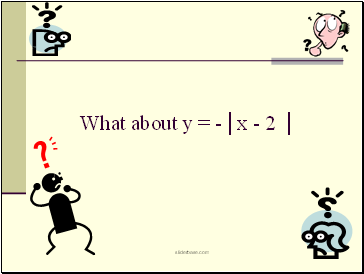What about y = -│x - 2 │

Slide 15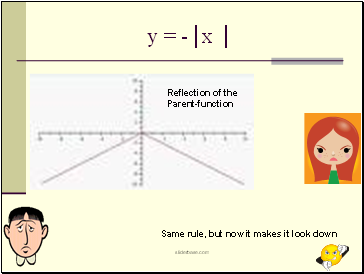y = -│x │

Same rule, but now it makes it look down

Reflection of the

Parent-function

Slide 16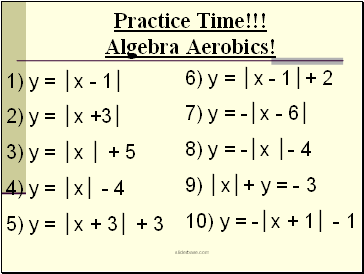Practice Time!!! Algebra Aerobics!

1) y = │x - 1│

2) y = │x +3│

3) y = │x │ + 5

4) y = │x│ - 4

5) y = │x + 3│ + 3

6) y = │x - 1│+ 2

7) y = -│x - 6│

8) y = -│x │- 4

9) │x│+ y = - 3

10) y = -│x + 1│ - 1

Slide 17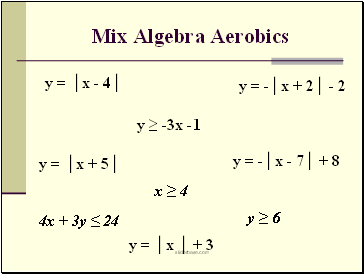Mix Algebra Aerobics

y = │x - 4│

y = │x + 5│

y = │x │ + 3

y = -│x + 2│ - 2

y = -│x - 7│ + 8

4x + 3y ≤ 24

y ≥ 6

x ≥ 4

y ≥ -3x -1

Slide 18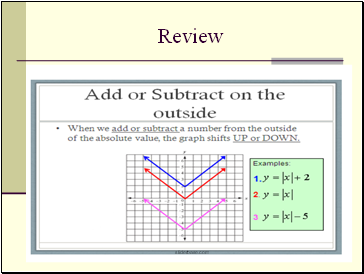Review

Slide 19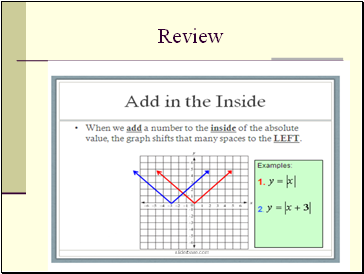Review

Slide 20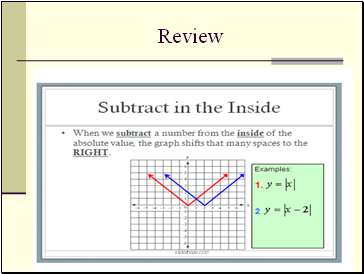Review

Slide 21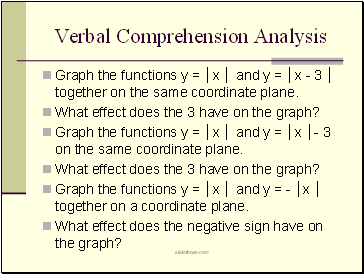## Verbal Comprehension Analysis

Graph the functions y = │x │ and y = │x - 3 │ together on the same coordinate plane.

What effect does the 3 have on the graph?

Graph the functions y = │x │ and y = │x │- 3 on the same coordinate plane.

What effect does the 3 have on the graph?

Graph the functions y = │x │ and y = - │x │ together on a coordinate plane.

What effect does the negative sign have on the graph?

Slide 22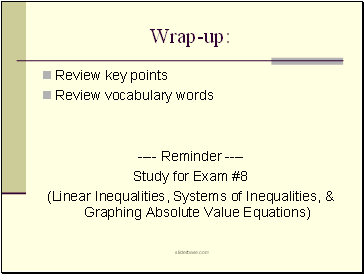## Wrap-up

Review key points

Review vocabulary words

---- Reminder ----

Study for Exam #8

(Linear Inequalities, Systems of Inequalities, & Graphing Absolute Value Equations)

Go to page:
1  2